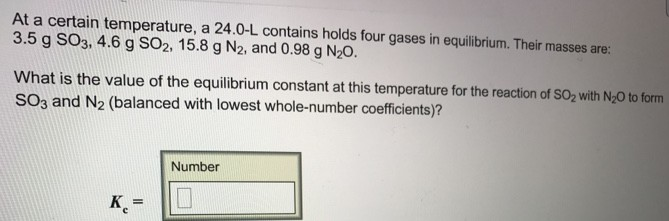Chemistry Practice Problems Equilibrium Expressions Practice Problems Solution: At a certain temperature a 24.0-L contains holds f...

🤓 Based on our data, we think this question is relevant for Professor Munoz's class at UTA.

# Solution: At a certain temperature a 24.0-L contains holds four gases in equilibrium. Their masses are: 3.5 g SO3, 4.6 g SO2, 15.8 g N2, and 0.98 g N2O. What is the value of the equilibrium constant at this temperature for the reaction of SO2 with N2O to form SO3 and N2 (balanced with lowest whole-number coefficients)?

###### Problem

At a certain temperature a 24.0-L contains holds four gases in equilibrium. Their masses are: 3.5 g SO3, 4.6 g SO2, 15.8 g N2, and 0.98 g N2O.

What is the value of the equilibrium constant at this temperature for the reaction of SO2 with N2O to form SO3 and N2 (balanced with lowest whole-number coefficients)?View Complete Written Solution

Equilibrium Expressions

Equilibrium Expressions

#### Q. Write the equilibrium constant expression for this reaction: H2C2O4 (aq) → 2H+ (aq) + C2O42- (aq)

Solved • Tue Aug 14 2018 14:26:14 GMT-0400 (EDT)

Equilibrium Expressions

#### Q. Consider the following reaction. CO(g) + H2O(g) ⇌ CO2(g) + H2(g) a. Write the expression for the equilibrium constant. b. Calculate K using the follow...

Solved • Tue Aug 14 2018 10:56:18 GMT-0400 (EDT)

Equilibrium Expressions

#### Q. Write an expression for the equilibrium constant for the reaction. 2 NO(g) + O2(g) ⇌ 2 NO2(g)

Solved • Tue Aug 14 2018 10:53:46 GMT-0400 (EDT)

Equilibrium Expressions

#### Q. The Keq for the reaction: A + B ⇌ AB is 7 What is the Keq for 3 AB ⇌ 3A + 3B?

Solved • Tue Aug 14 2018 10:52:26 GMT-0400 (EDT)